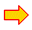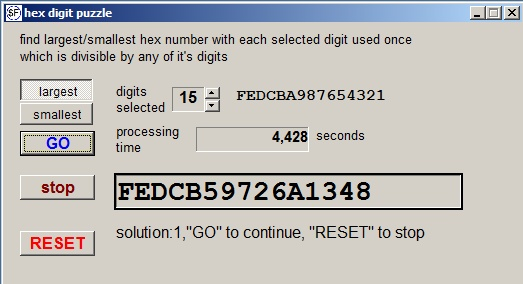A hexadecimal digit puzzleQuestion:
Find hexadecimal numbers that show a selected digit once and where each digit divides the number.

This little Delphi-project helps.download Delphi projectdownload program

Below is the program at work:Method
The number is incremeneted / decremented by the LCM (least common multiple) of the selected digits.
A check for recurrence of digits is done by examining the 4 bit hex groups in the number.
The number is of format Int64.
Because shift operations do not work well with 64 bit integers, the number is also split in two 32 bit integers.

When all numbers are examined, a new combination of digits is chosen.